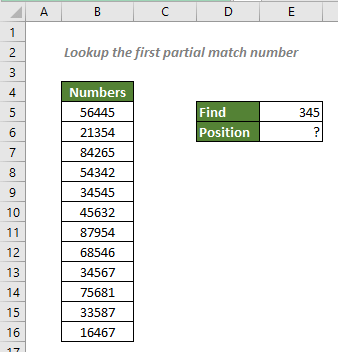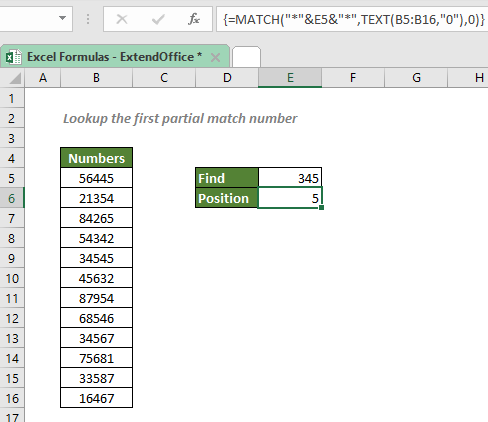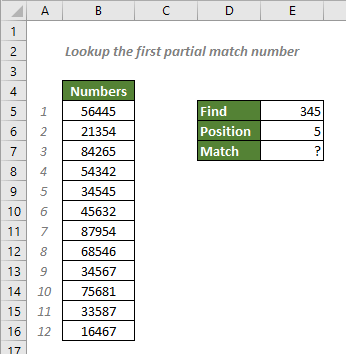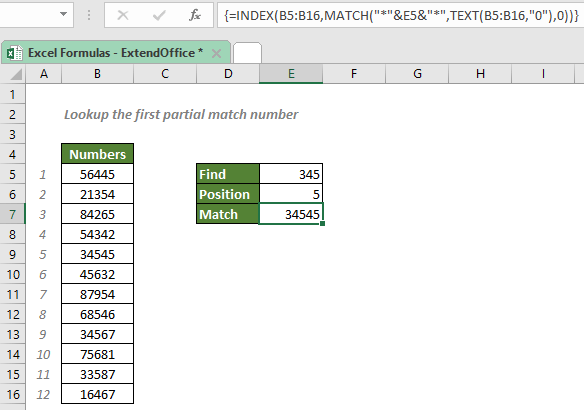## Lookup the first partial match number

There are cases that you need to get the position of the first partial match that contains specific number in a range of numeric values in Excel. In this case, a MATCH and TEXT formula that incorporates asterisk (*), the wildcard that matches any number of characters, will do you a favor. And if you also need to know the exact value at that position, you can add the INDEX function to the formula.#### Get the position of the first partial match number

To get the position of the first partial match number containing “345” from the number range as shown above, a MATCH and TEXT formula together with wildcards will help you in this way: In order to match any numbers that contain 345, you will wrap the number 345 between two asterisks (*). However, the operation will convert the numeric value to a text value. So, you will have to use the TEXT function to convert numbers in the number range to text. Only in this case, the MATCH function will be able to find the position of the partial match properly.

#### Generic syntax

=MATCH("*"&number&"*",TEXT(lookup_array,"0"),0)

√ Note: This is an array formula that requires you to enter with Ctrl + Shift + Enter.

• number: The number you specified to lookup its first partial match.
• lookup_array: The range of numeric values to retrieve the position of the first partial match from.

To get the position of the first match number that contains “345”, please copy or enter the formulas below in the cell E6, and press Ctrl + Shift + Enter to get the result:

=MATCH("*"&345&"*",TEXT(B5:B16,"0"),0)

Or, use a cell reference to make the formula dynamic:

=MATCH("*"&E5&"*",TEXT(B5:B16,"0"),0)

√ Note: To stick a cell reference/number and text together, you must add an ampersand (&) in between them. And the text should be enclosed in double quotes.#### Explanation of the formula

=INDEX("*"&345&"*",TEXT(B5:B16,"0"),0)

• TEXT(B5:B16,"0"): The TEXT function converts all the numeric values in B5:B16 to text with the format code “0”. So, we will get a text array like this: {"56445";"21354";"84265";"54342";"34545";"45632";"87954";"68546";"34567";"75681";"33587";"16467"}.
• INDEX("*"&345&"*",TEXT(B5:B16,"0"),0) = INDEX("*"&345&"*",{"56445";"21354";"84265";"54342";"34545";"45632";"87954";"68546";"34567";"75681";"33587";"16467"},0): The lookup value "*"&345&"*" can match any text strings that contain the string “345”, no matter what position 345 is in the text strings. The match_type 0 asks the MATCH function to find the position of the first exact lookup value in the array. So, the MATCH will return 5.

#### Retrieve the first partial match number

To retrieve the first partial match number based on the position provided by MATCH as shown below, we can put a spotlight on the INDEX function.#### Generic syntax

=INDEX(MATCH(return_range,"*"&number&"*",TEXT(lookup_array,"0"),0))

√ Note: This is an array formula that requires you to enter with Ctrl + Shift + Enter.

• return_range: The range where you want the combination formula to return the first partial match from.
• number: The number you specified to lookup its first partial match.
• lookup_array: The range of numeric values to retrieve the first partial match from.

To get the first match number that contains “345”, please copy or enter the formulas below in the cell E7, and press Ctrl + Shift + Enter to get the result:

=INDEX(MATCH(B5:B16,"*"&345&"*",TEXT(B5:B16,"0"),0))

Or, use a cell reference to make the formula dynamic:

=INDEX(MATCH(B5:B16,"*"&E5&"*",TEXT(B5:B16,"0"),0))#### Explanation of the formula

=INDEX(B5:B16,MATCH("*"&E5&"*",TEXT(B5:B16,"0"),0))

• MATCH("*"&E5&"*",TEXT(B5:B16,"0"),0) = 5:
• INDEX(B5:B16,MATCH("*"&E5&"*",TEXT(B5:B16,"0"),0)) = INDEX(B5:B16,5): The INDEX function returns the 5th value in the return range B5:B16, which is 34545.

#### Related functions

Excel MATCH function

The Excel MATCH function searches for a specific value in a range of cells, and returns the relative position of the value.

Excel TEXT function

The TEXT function converts a value to text with a specified format in Excel.

Excel INDEX function

The Excel INDEX function returns the displayed value based on a given position from a range or an array.

#### Related Formulas

Locate first partial match with wildcards

To get the position of the first partial match that contains specific text string in a range in Excel, you can use a MATCH formula with wildcard characters - the asterisk (*) and question mark (?).

Lookup closest match

To look for the closest match of a lookup value in a numeric dataset in Excel, you can use the INDEX, MATCH, ABS and MIN functions together.

Lookup closest match value with multiple criteria

In some cases, you may need to lookup the closest or approximate match value based on more than one criteria. With the combination of INDEX, MATCH and IF functions, you can quickly get it done in Excel.

Two-way approximate match with multiple criteria

In this tutorial, we will talk about how to look for an approximate match based on multiple criteria listed both in columns and rows in an Excel spreadsheet, with the help of INDEX, MATCH, and IF functions.

### The Best Office Productivity Tools

#### Kutools for Excel - Helps You To Stand Out From Crowd

 Popular Features: Find, Highlight or Identify Duplicates  |  Delete Blank Rows  |  Combine Columns or Cells without Losing Data  |  Round without Formula ... Super VLookup: Multiple Criteria  |  Multiple Value  |  Across Multi-Sheets  |  Fuzzy Lookup... Adv. Drop-down List: Easy Drop Down List  |  Dependent Drop Down List  |  Multi-select Drop Down List... Column Manager: Add a Specific Number of Columns  |  Move Columns  |  Toggle Visibility Status of Hidden Columns  |  Compare Columns to Select Same & Different Cells ... Featured Features: Grid Focus  |  Design View  |  Big Formula Bar  |  Workbook & Sheet Manager | Resource Library (Auto Text)  |  Date Picker  |  Combine Worksheets  |  Encrypt/Decrypt Cells  |  Send Emails by List  |  Super Filter  |  Special Filter (filter bold/italic/strikethrough...) ... Top 15 Toolsets:  12 Text Tools (Add Text, Remove Characters ...)  |  50+ Chart Types (Gantt Chart ...)  |  40+ Practical Formulas (Calculate age based on birthday ...)  |  19 Insertion Tools (Insert QR Code, Insert Picture from Path ...)  |  12 Conversion Tools (Numbers to Words, Currency Conversion ...)  |  7 Merge & Split Tools (Advanced Combine Rows, Split Excel Cells ...)  |  ... and more

Kutools for Excel Boasts Over 300 Features, Ensuring That What You Need is Just A Click Away...#### Office Tab - Enable Tabbed Reading and Editing in Microsoft Office (include Excel)

• One second to switch between dozens of open documents!
• Reduce hundreds of mouse clicks for you every day, say goodbye to mouse hand.
• Increases your productivity by 50% when viewing and editing multiple documents.
• Brings Efficient Tabs to Office (include Excel), Just Like Chrome, Edge and Firefox.